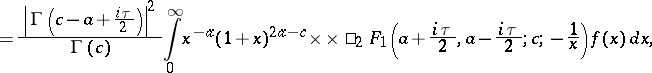# Olevskii transform

(diff) ← Older revision | Latest revision (diff) | Newer revision → (diff)

\begin{equation} \tag{a1} F ( \tau ) = \end{equation}where $\square _ { 2 } F _ { 1 } ( a , b ; c ; z )$ is a Gauss hypergeometric function. It was introduced by M.N. Olevskii in [a1].

Letting $a = 1 / 2$, one obtains the Mehler–Fock transform. By changing the variable $x = \operatorname { sinh } ^ { - 2 } t$ and the respective parameters of the Gauss function, one obtains the Fourier–Jacobi transform [a2].

One can show that the Olevskii transform is the composition of the Kontorovich–Lebedev transform and the Hankel transform (cf. Integral transform; Hardy transform).

The Gauss function in the integral (a1) is the hypergeometric series for $x > 1$ and for $0 < x \leq 1$ one can understand it as an analytic continuation, which can be obtained from the Mellin–Barnes integral representation [a3].

The following integral transform is also called the Olevskii transform. It is an integral over the index $\tau$ of the Gauss function,

\begin{equation} \tag{a2} F ( x ) = \frac { x ^ { - a } ( 1 + x ) ^ { 2 a - c } } { \Gamma ( c ) } \times \end{equation}

\begin{equation*} \times \int _ { - \infty } ^ { \infty } \tau \left| \Gamma \left( c - a + \frac { i \tau } { 2 } \right) \right| ^ { 2 } \times \times \square _ { 2 } F _ { 1 } \left( a + \frac { i \tau } { 2 } , a - \frac { i \tau } { 2 } ; c ; - \frac { 1 } { x } \right) f ( \tau ) d \tau. \end{equation*}

Here, $f$ is an arbitrary odd function belonging to the space $L _ { 2 } ( \mathbf{R} ; \omega ( \tau ) )$, where

\begin{equation*} \omega ( \tau ) = \frac { \tau } { \operatorname { sinh } ( \pi \tau ) } \left| \frac { \Gamma ( c - a + \frac { i \tau } { 2 } ) } { \Gamma ( a + \frac { i \tau } { 2 } ) } \right| ^ { 2 } . \end{equation*}

The transform (a2) maps this space onto the space $L _ { 2 } ( \mathbf{R} _ { + } ; x ^ { - 1 } ( 1 + x ) ^ { c - 2 a } )$ and the Parseval equality holds:

\begin{equation*} \int _ { 0 } ^ { \infty } | F ( x ) | ^ { 2 } ( 1 + x ) ^ { c - 2 a } \frac { d x } { x } = \end{equation*}

\begin{equation*} = 8 \pi ^ { 2 } \int _ { - \infty } ^ { \infty } \tau \operatorname { sinh } ( \pi \tau ) \left| \frac { \Gamma ( c - a + \frac { i \tau } { 2 } ) } { \Gamma ( a + \frac { i \tau } { 2 } ) } | ^ { 2 } \right| f ( \tau ) | ^ { 2 } d \tau. \end{equation*}

How to Cite This Entry:
Olevskii transform. Encyclopedia of Mathematics. URL: http://encyclopediaofmath.org/index.php?title=Olevskii_transform&oldid=50844
This article was adapted from an original article by S.B. Yakubovich (originator), which appeared in Encyclopedia of Mathematics - ISBN 1402006098. See original article